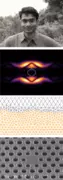X
Electromagnetic Field Imaging in Arbitrary Scattering Environments
Sastry K., Bhat C., Solimene R.
Published in IEEE
2021
Volume: 7

Pages: 224 - 233
Abstract
In this article, we propose a method to reconstruct the total electromagnetic field in an arbitrary two-dimensional scattering environment without any prior knowledge of the incident field or the permittivities of the scatterers. However, we assume that the region between the scatterers is homogeneous and that the approximate geometry describing the environment is known. Our approach uses field measurements and a compressive sensing inspired algorithm to estimate the incident field and the tangential electric and magnetic fields on the scatterers' surfaces. These estimates are then used to predict the field everywhere using Huygens' principle. Further, we identify the best measurement locations in the environment, which reduces the estimation error to approximately half of the error obtained when using random locations. We show that in an indoor scenario with up to four scattering objects, the total electric field is recovered with less than 10% error when the number of measurements is just 0.3 times the number of unknowns in which the problem is formulated. The formulated problem is solved using 'Total field - Compressive sensing based subspace optimization method' - an algorithm that leverages the sparsity of the tangential fields in known transformation domains to obtain an optimal solution. © 2015 IEEE.
•